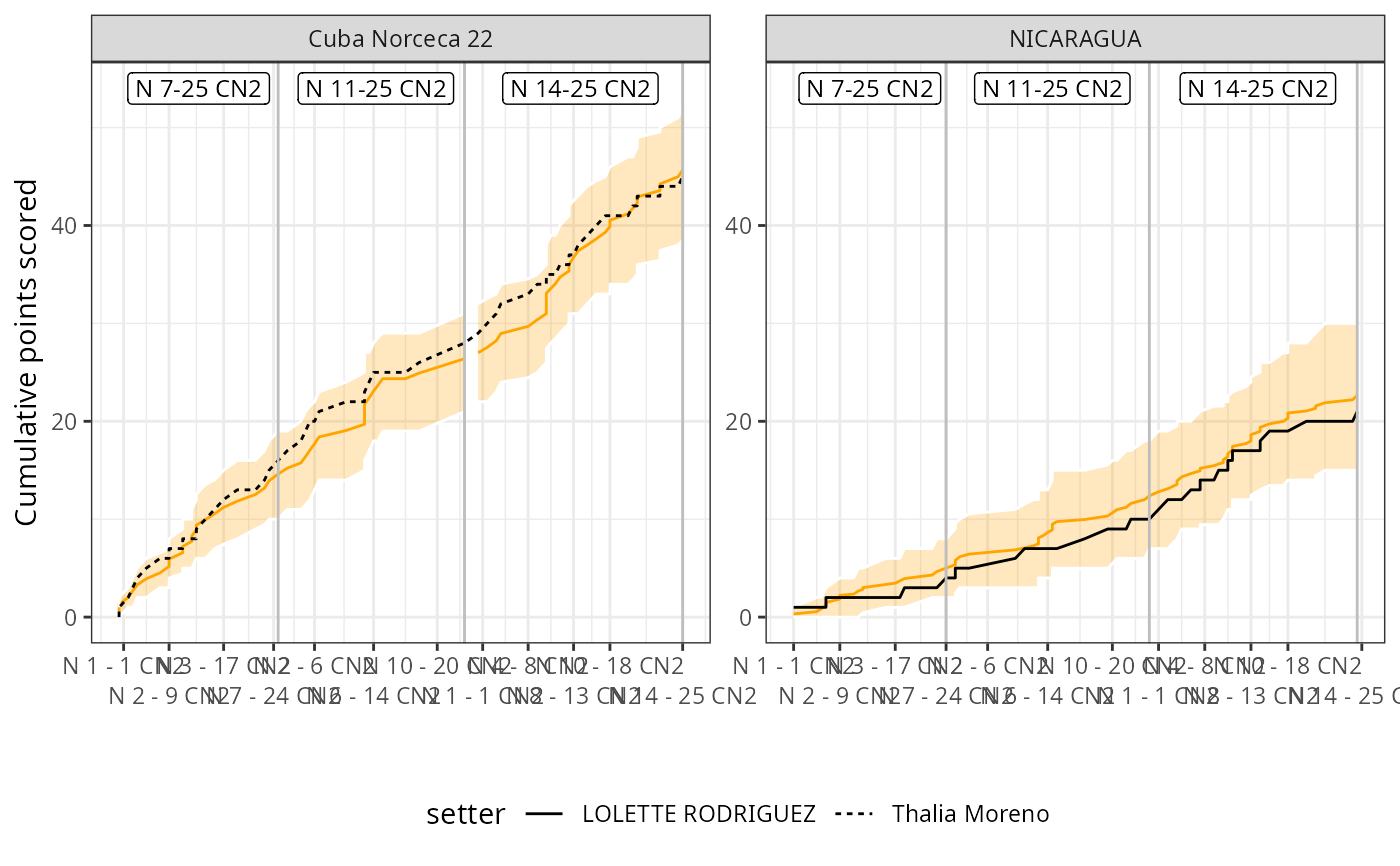Plot a simulated setter distribution

## Usage

ov_plot_ssd(
ssd,
overlay_set_number = FALSE,
label_setters_by = "name",
font_size = 11
)

## Arguments

ssd

simulated setter distribution output as returned by ov_simulate_setter_distribution()

overlay_set_number

boolean: if TRUE, overlay set number and score in the plot

label_setters_by

string: either "id" or "name"

font_size

numeric: font size

## Examples

dvw <- ovdata_example("190301_kats_beds")
setter <- ov_simulate_setter_distribution(dvw = dvw,
n_sim = 150, attack_by = "zone")
ov_plot_ssd(setter, overlay_set_number = TRUE)